# An emphasis on Graphene based junction devices

N/A
N/A
Protected

Share "An emphasis on Graphene based junction devices"

Copied!
172
0
0

Full text

The presence of spin-orbit couplings, namely intrinsic and Rashba couplings in graphene, is included via the Kane-Mele Hamiltonian. The transport properties and practical applications of Kane-Mele normal insulators and superconductors are thoroughly investigated.

## Spin-orbit coupling

In the next section we briefly show a more thorough technique to obtain the spin-orbit coupling, starting with relativistic quantum mechanics for completeness. There may be three reasons behind the origin of spin-orbit coupling in material. a) Impurities in the conductive layer, the main source of SOC in metallic systems.

## Rashba spin-orbit coupling

If an electron moves along the x direction, the spin order of the eigenvector becomes (1;±i), that is, spin up and spin down are locked in the y direction. Fig.(1.1d) represents the energy spectrum of an electron in the presence of a magnetic field B, the spin degeneracy is canceled by the Zeeman~ splitting, and the gap separating the spin-up and spin-down bands is equal to gµBB.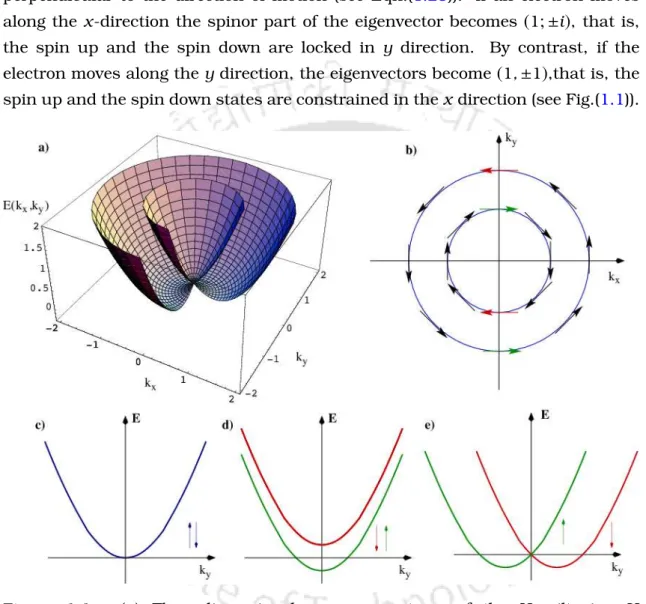Figure 1.1: (a) Three-dimensional energy spectrum of the Hamiltonian H (Eqn.(1.21)). (b) The Fermi energy contours for the Hamiltonian H

## Bogoliubov-deGennes equations

Fig. (1.1e) presents a one-dimensional view of the energy spectrum for an electron in the presence of RSOC. E0 is the ground state energy and ϸn is the excitation energy of the quasiparticle in the n state.

## Normal metal and superconductor junction: Andreev reflection

Here we will specify how the components of the velocity change according to various processes taking place at the interface. iii)Transmission as electron: v⊥→v⊥, vk→ vk. But in a NIS transition, the low energy transmission is strongly suppressed due to the specular reflection.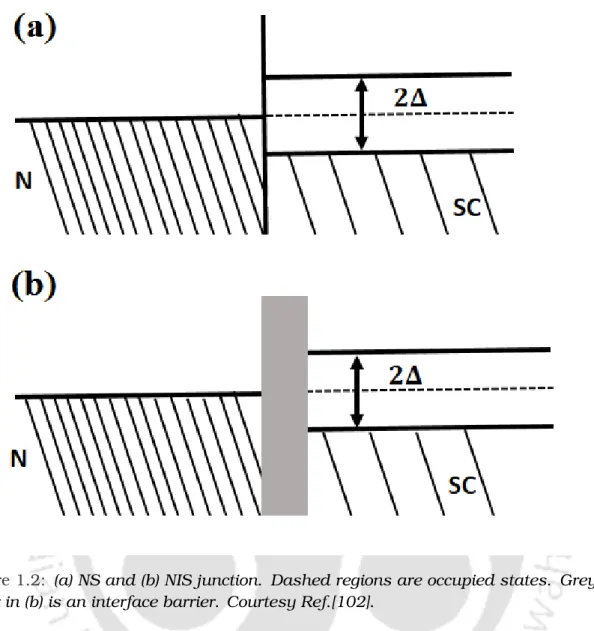Figure 1.2: (a) NS and (b) NIS junction. Dashed regions are occupied states. Grey block in (b) is an interface barrier

## Normal-insulator-superconductor junction and

Further, if we adjust the distance between the surfaces and change the wavelength of the light, the same oscillations in the intensity spectrum of the transmitted light can be obtained. Quantum interference occurs when such a round trip becomes a multiple of the electron's wavelength.

## Basics of Graphene

Each time an electron reaches one of the surfaces, it can bounce back and can also be emitted. So even in this case, if we change the width of the insulating layer or the alternating wavelength of the electron, the conductivity will show fluctuations.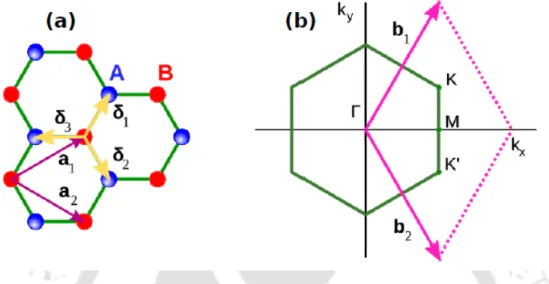Figure 1.7: (a) Honeycomb lattice structure of graphene. The vectors ~ δ 1 , ~ δ 2 and ~ δ 3

## Klein tunneling in graphene

Only two of these six points are unequal and they are usually referred to as the K and K0 points. As in this thesis, the conduction properties of the graphene-based NIS (although the insulating layer is modeled in a different way) junction has been studied, here we will briefly discuss the Klein tunneling phenomenon in graphene.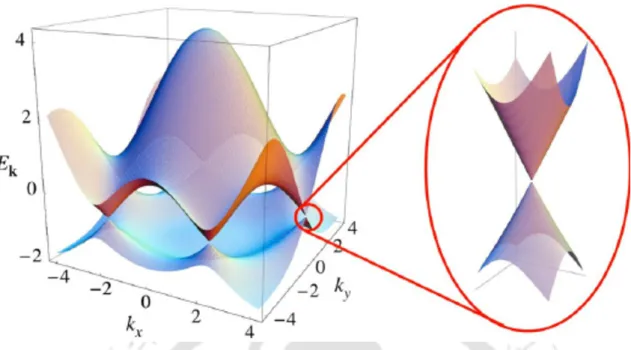Figure 1.8: Electronic band structure of graphene. Left: energy spectrum (in units of nearest neighbour hopping strength, t 1 )

## Kane-Mele Model

As we want to study the transport properties of graphene-based junction devices in the presence of spin-orbit interactions, we have considered a Kane-Mele model in the presence of Rashba and intrinsic spin-orbit interactions to calculate the tunneling conductance and properties thermoelectric. of graphene-based coupling devices.

## Outline of the thesis

The momenta of the electrons and the holes in the normal region, kN1 and kN2 are given by,. Hieraσ and bσ denote the amplitudes of the Andreev reflection (AR) and normal reflection (NR), respectively.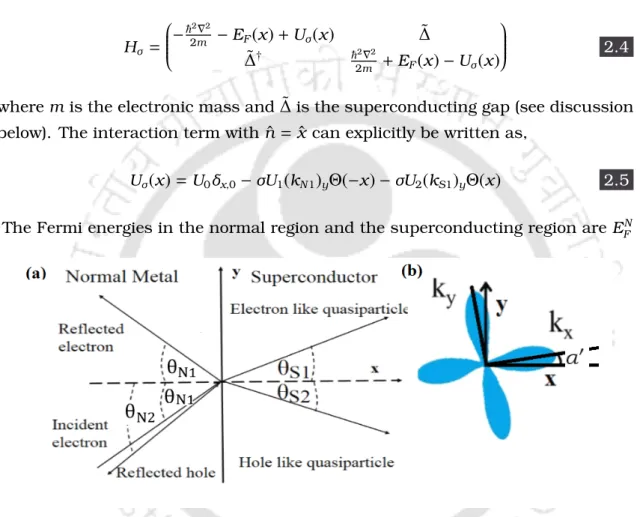Figure 2.1: (a) Schematic illustration of the reflection and the transmission pro- pro-cesses of the quasiparticle at an NS junction

## Normal-insulator-superconductor (NIS) junction

Here aσ and bσ denote the amplitude of pinhole reflection (AR) and normal reflection (NR) respectively in the normal region. Further, pσ and qσ represent the amplitudes of the incoming and reflected holes in the insulating region.

## Graphene based NS junction: Kane-Mele model

In the graph near the KandK0 points, the energy dissipation is a linear function of momentum, where the K and K0 points are given by,. The expressions for momenta corresponding to all of them are given by the following compact form, .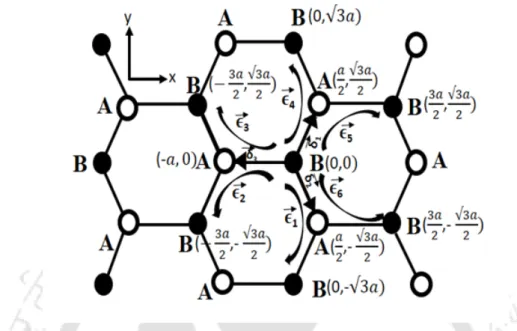Figure 2.4: Schematic diagram of graphene. The coordinates of A and B sublattices are shown

## Graphene (Kane-Mele) based NIS junction

Since the momentum parallel to the interface is conserved during the tunneling process, we can write: Furthermore, in the tunneling conduction of Sr2RuO4 , the presence of broad subgap peaks can also be described in terms of the surface ABS, which usually occurs in the p-wave type chiral superconductor [125,126].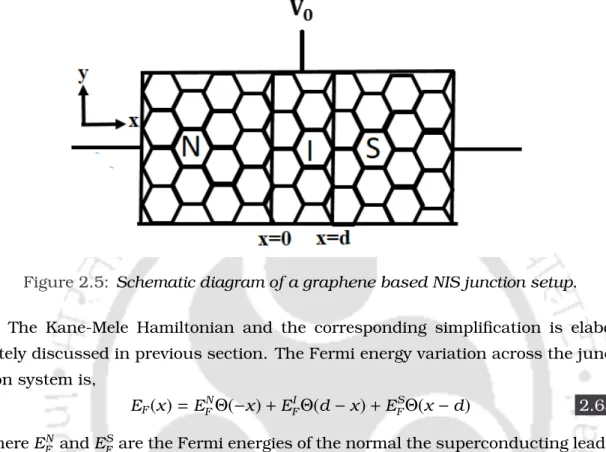Figure 2.5: Schematic diagram of a graphene based NIS junction setup.

## Results on s-wave pairing symmetry

Thus, we show the variation of the amplitude of AR and NR as the function of the bias energy in Figure (3.2). Therefore, we show the variation of the zero-bias conductance, as a function of the Rashba strength, λRin figure (3.5).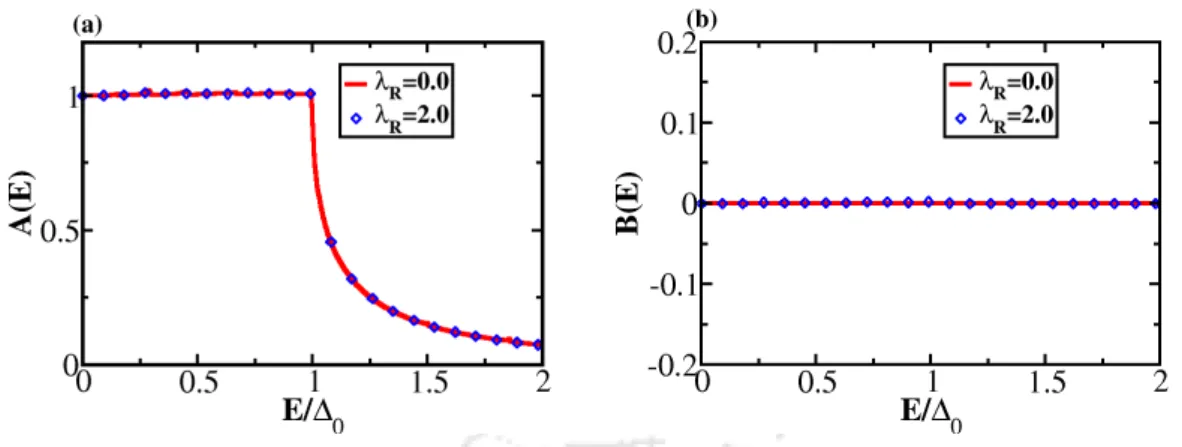Figure 3.2: The variation of the AR in (a) and NR in (b) for s-wave superconductor as a function of E/ ∆ 0 for a transparent barrier.

## Other pairing symmetries

Again, for an opaque barrier, with the addition of RSOC, the amplitude of AR increases and the amplitude of NR decreases. Furthermore, it is clear that, with the inclusion of RSOC, the surface ABS is not affected, which is the hallmark of the p-wave pair symmetry.

Fig.(3.6a) shows that for transparent case with the inclusion of Rashba spin-orbit coupling there is no change in conductance although the momenta of the electrons and holes are modified. Here we get a maximum value at E =0 due to the fact that the contribution of the AR is maximum at E = ∆0.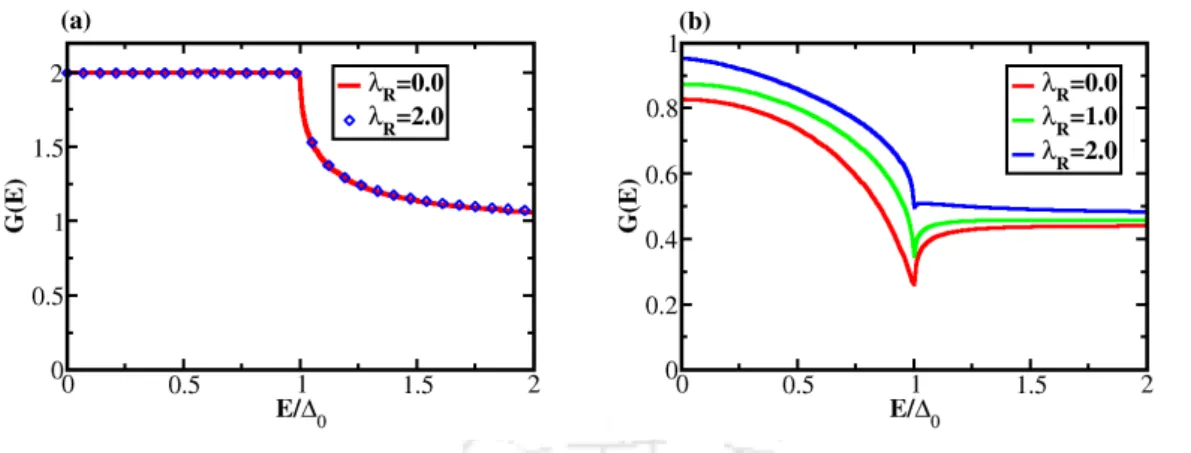Figure 3.6: The variation of the conductance, G for a p-wave superconductor as a function of E/ ∆ 0 for different strengths of RSOC for (a) U 0 = 0 (transparent barrier) and (b) U 0 = E F N (opaque barrier).

## Fermi surface mismatch

Therefore, for the above conditions, no transmission will take place to the superconducting region and will therefore not contribute to the conduction. With the addition of RSOC, the conductivity for the transparent case decreases and for the opaque barrier the conductivity increases.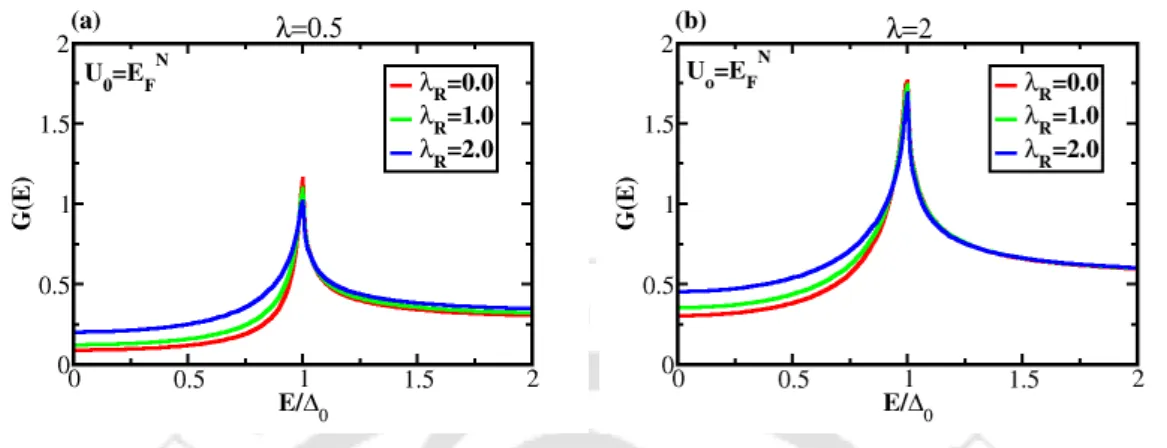Figure 3.9: The variation of the conductance, G ( E ) for a s-wave superconductor as a function of E/ ∆ 0 for (a) λ = 0.5, (b) λ = 2 with an opaque barrier (U 0 = E F N ).

With the introduction of finite quasiparticle lifetimes, all expressions containing energy, E are renormalized as mentioned above and then they must be inserted into the corresponding equations to calculate the conductivity. With the introduction of finite quasiparticle lifetimes, the dependence of the conductance spectrum on RSOC does not change.

## Normal-superconductor junction (NS) with interfacial RSOC

It should not be obvious how an inclusion of Γ(orτQP) can interfere with the RSOC present in the metallic lead and thus whether it helps or hinders the low energy conduction properties of an NS junction. Comparison with Fig.

## Normal-insulator-superconductor junction

### Results and discussions

Now we show the conductance as a function of bias, E, (in units of the superconducting gap, that is, E/∆0) for different values ​​of RSOC for two different ranges of the effective barrier potential, χ1 and χ2 in Fig. .(3.14). So the dependence of the tunneling conductance on the RSOC strength is crucially dependent on the effective barrier potential.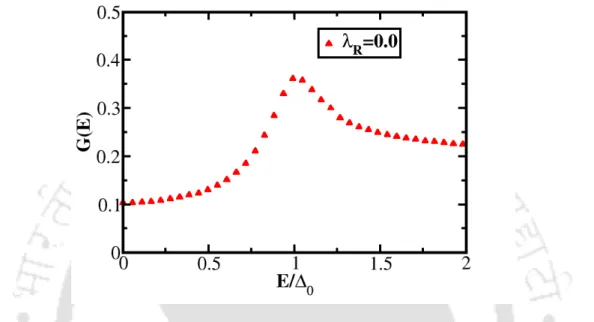Figure 3.12: The variation of the conductance, G for a s-wave superconductor as a function of biasing energy, E/ ∆ 0 for a conventional NIS junction.

## Conclusions

### Seebeck coefficient

Importantly, the magnitude and sign of the Seebeck coefficient quantifies the asymmetry of the electron distribution near the Fermi level. A first-order expansion of the Fermi-Dirac distribution function in (dI)T and (dI)V gives the following expression for the Seebeck coefficient,S,.

### Figure of Merit

We can conclude that δV arises due to the Seebeck effect due to the temperature difference that exists between the junctions. We note that KT and KV indicate the thermal conductivity due to the temperature difference, δT, and the voltage difference, δV.

### Thermoelectric cooling

JNS(EFN, TN;EFS, TS)+INS(EFN, TN;EFS, TS)VB =JSN(EFN, TN;EFS, TS) 4.21 As, in our calculations we shifted energies by the Fermi energies of the respective electrodes, the final form of the thermal currents is given by, . The performance of this junction as a self-cooling device can be measured by the coefficient of performance (COP) where COP is defined as the ratio of the heat removed from the cold reservoir to the electrical power required to power the system drive.

## Results and discussions: Thermopower

### Figure of Merit

Fig.(4.6) shows the value as a function of the barrier potential for different values ​​of the strength of the Rashba coupling, the temperature being fixed at T =0.3∆0. Further, the variation of the figure of merit as a function of both Rashba spin-orbit coupling and x is shown in Fig. (4.8b).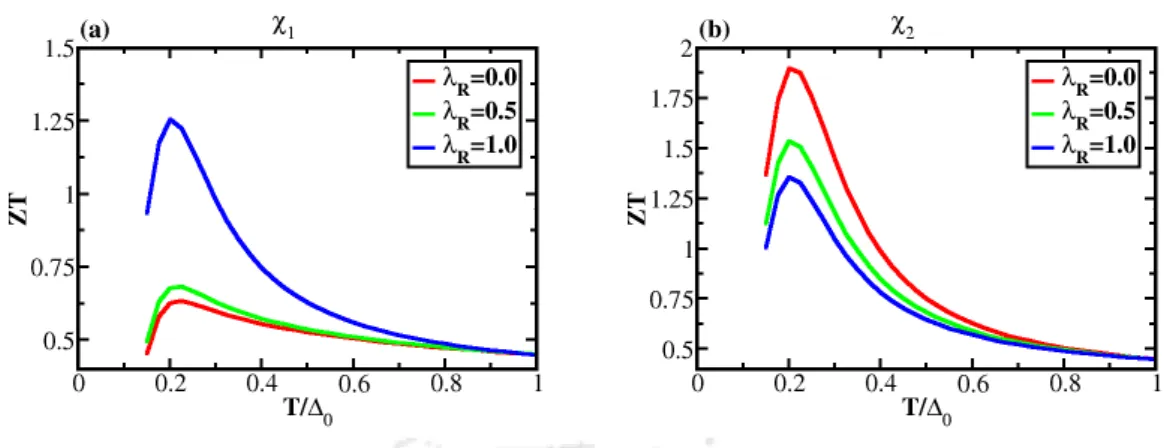Figure 4.7: The variation of the Figure of Merit (ZT ) as a function of temperature, T/ ∆ 0 for two different regions of the effective barrier potential, χ, namely, (a) χ 1 , (b) χ 2 .

## Results and discussions: Thermoelectric cooling

### Coefficient of Performance (COP)

Fig.(4.12) shows the COP as a function of the effective barrier potential (χ) for different values ​​of RSOC, where bias is fixed at VB= 0.15∆0. In fig. (4.13) we present the variation of COPas a function of the bias for two different values ​​of the effective barrier potential, χ (namely some χ1 and χ2).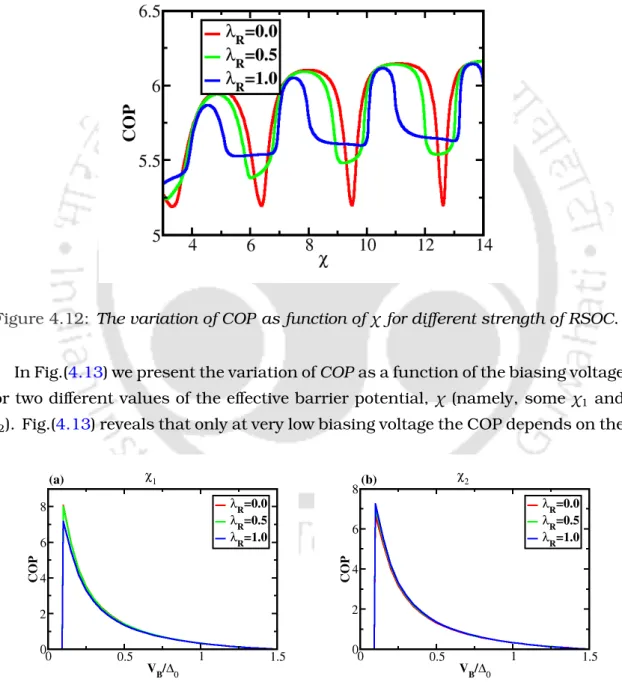Figure 4.12: The variation of COP as function of χ for different strength of RSOC.

## Concluding remarks

### Results and Discussions on Kane-Mele NS junction

In Fig. (5.2) we have shown the conductivity as a function of the strength of the RSOC for different rotations. Conductivity as a function of ISOC strength (in the absence of RSOC) is shown in Fig. (5.4) where we have assumed a zero bias condition.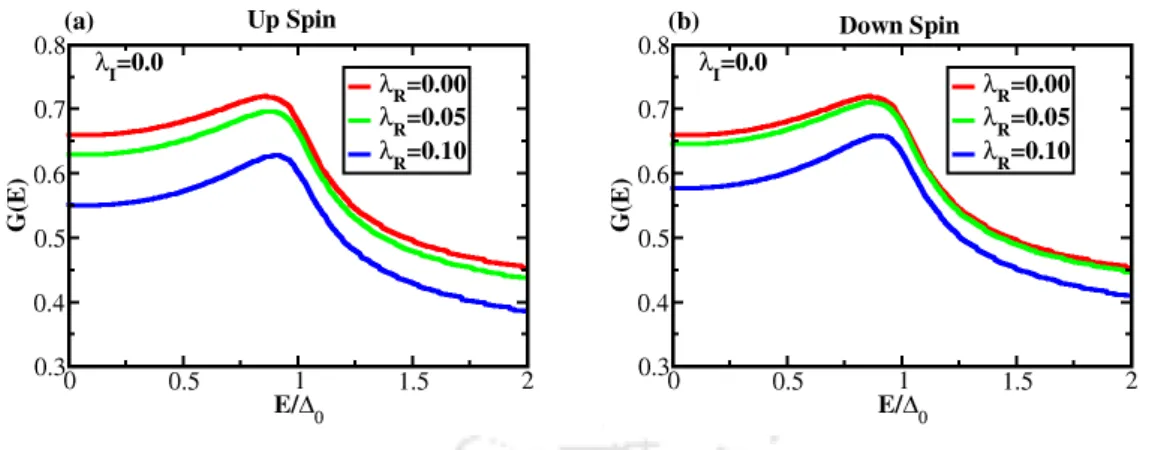Figure 5.1: (a) The variation of conductance for the up spin, G up as a function of biasing voltage (scaled by the superconducting order parameter), E/ ∆ 0 , (b) The variation of conductance for the down spin, G down as the function of E/ ∆ 0 .

## Kane-Mele NIS junction

### Results and Discussions on Kane-Mele NIS junction

In Fig.(5.7), the spin-polarized conductance, Gσ is shown as a function of the effective barrier potential, χ, while the strength of the RSOC term is varied with the strength of the intrinsic term held to zero. The conduction characteristics of the full Kane-Mele junction as a function of χ are shown in Fig.(5.9).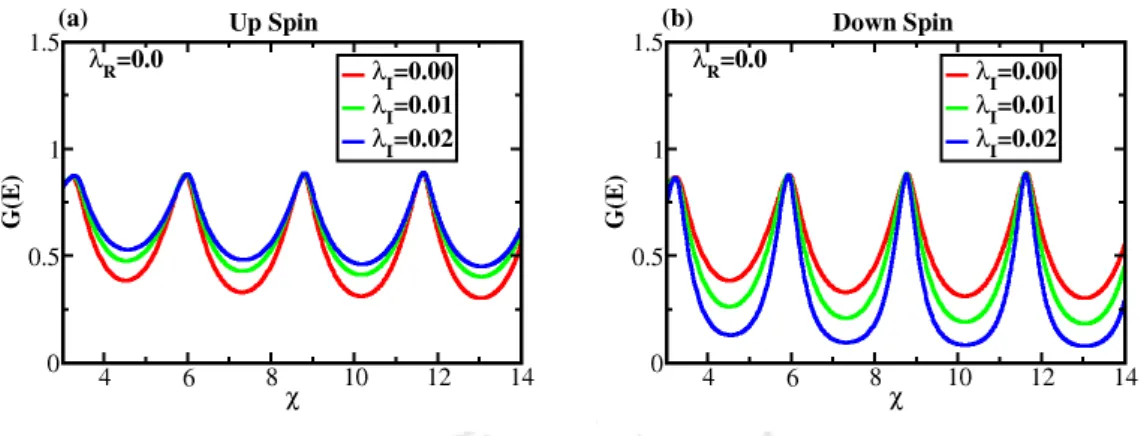Figure 5.8: The variation of conductance for (a) the up spin, G up and for (b) the down spin, G down as a function of χ with λ R = 0.

## Conclusion

### Seebeck coefficient

The population of electrons in the left and right wires is described by the Fermi-Dirac distribution function, fN and fS, respectively, where EFN =EFS at zero external bias. In the next subsection, we will discuss how the thermal current and the thermal conductivity can be calculated.

### Thermoelectric cooling

To get a clear idea of ​​the efficiency, one should calculate the spin-dependent FM, which is given by The performance of this compound as a self-cooling device can be measured by the coefficient of performance (COP), where COP is defined as the ratio of the heat removed from the cold reservoir to the electrical power needed to operate the system.

## Results and Discussions

### Seebeck Coefficient and Figure of Merit

The variation of the Seebeck coefficient,S as a function of the temperature (in units of superconducting gap, ∆0) for a pristine graphene is shown in Fig.(6.1a). The variation of the Figure of Merit, ZT as a function of the temperature (in units of superconducting gap, ∆0) is shown in Fig.(6.5).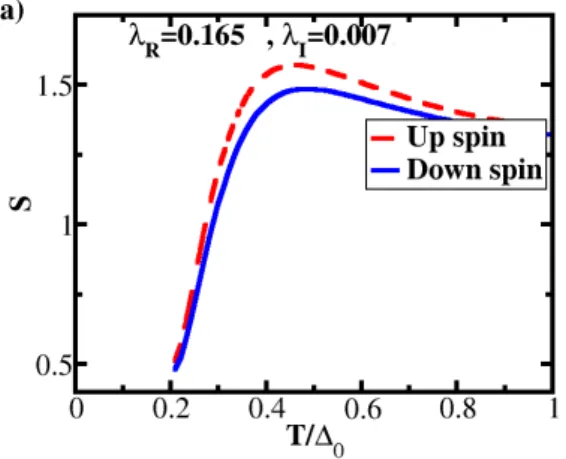Figure 6.2: The variation of the Seebeck coefficient, S as a function of temperature, T/ ∆ 0 for a larger RSOC parameter by one order greater magnitude compared to that of the Au decorated graphene.

### Thermoelectric cooling and coefficient of performance

For higher values ​​of the RSOC parameter, the thermoelectric cooling becomes smaller for both turns. The color plots provide the idea of ​​the range of values ​​of the RSOC, the ISOC parameter and the corresponding coefficient of performance.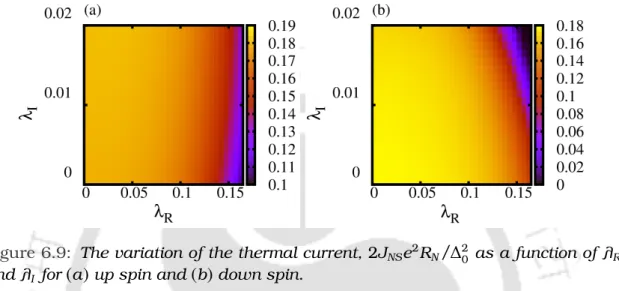Figure 6.9: The variation of the thermal current, 2J NS e 2 R N / ∆ 2 0 as a function of λ R and λ I for ( a ) up spin and ( b ) down spin.

## Conclusion

The variation of the conductance as a function of the effective barrier potential, χ for generic and graphene-based junction systems, is shown in Figure (7.2a) and Figure (7.2b), respectively. The Fermi energy of the superconducting lead has been considered as EFS = EFN (no Fermi surface mismatch).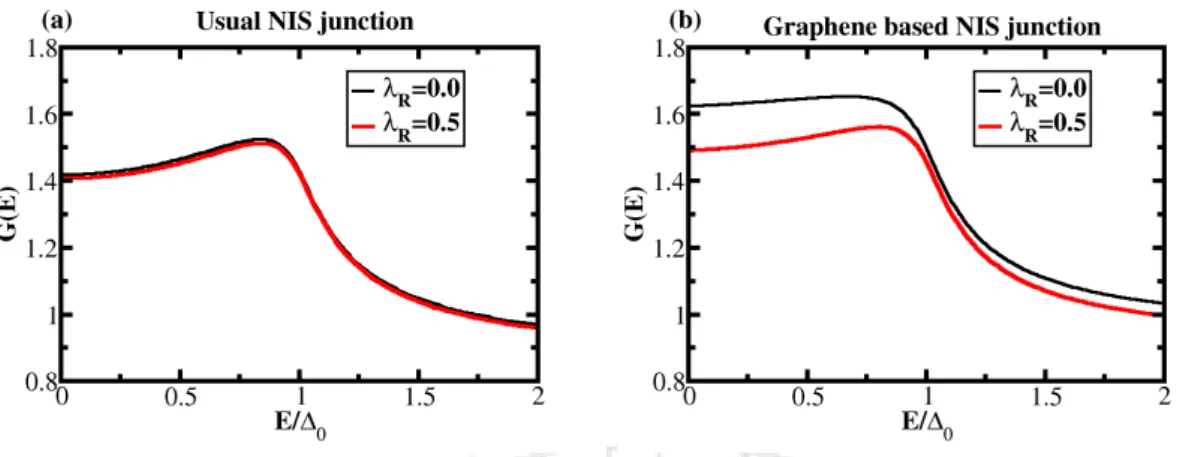Figure 7.1: The variation of the conductance, G as a function of biasing energy, E (scaled by superconducting order parameter, ∆ 0 ) for ( a ) usual NIS junction system and for ( b ) graphene based junction system

## Comparison of thermoelectric properties

Both the change in total conductivity as a function of bias energy shows a double peak structure (see Fig. (7.2)). Again, we took into account a certain value of the effective barrier potential, where the heat flow has a maximum value.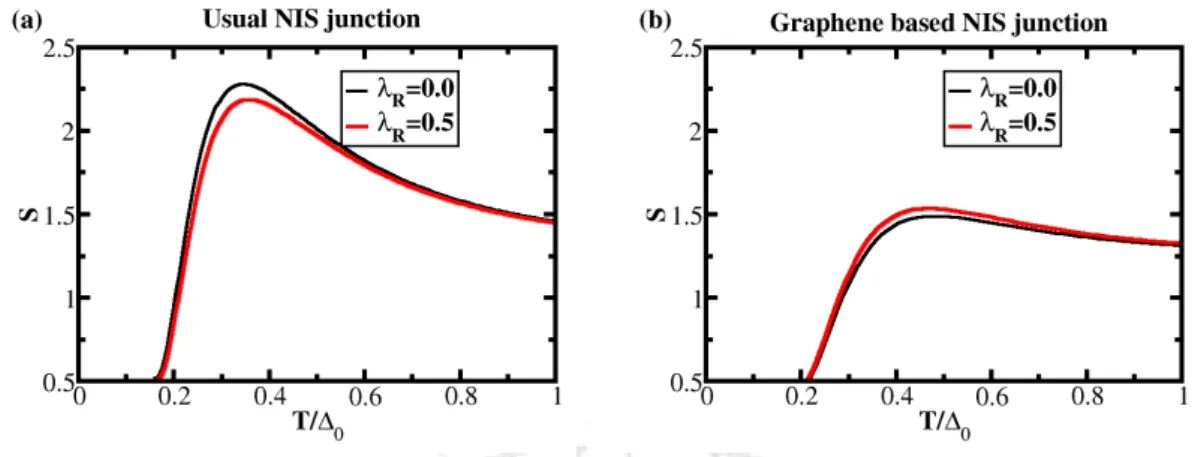Figure 7.3: The variation of the Seebeck coefficient , S as a function of temperature, T/ ∆ 0 (scaled by superconducting order parameter, ∆ 0 ) for ( a ) generic 2D junction system (parabolic dispersion) and for ( b ) graphene based junction system (linear

## Conclusion

We observed a Fabry-Perot-like oscillation for the tunneling conductance spectrum as a function of the effective barrier potential (the product of both V0 and the width, d). We observe the oscillation of the tunneling conductance spectrum as a function of the effective barrier potential.

Figure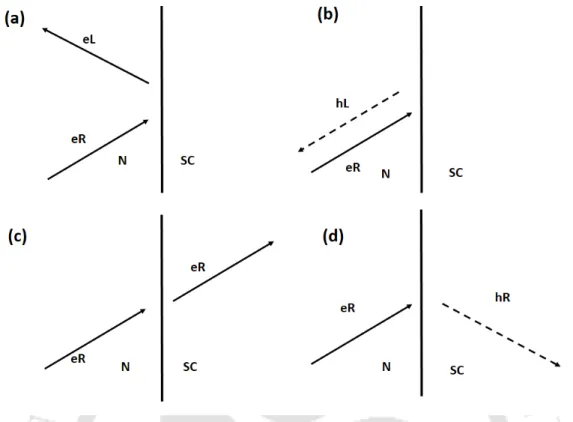+7

References

Related documents

Aiyagari Ramesh Thesis Submitted to the Department/ Center : Biosciences and Bioengineering Date of completion of Thesis Viva-Voce Exam : 22 November 2022 Key words for

2 hrs 2.5 Theory of organizational structures - nature and consequence of structure 2 hrs 3 Module B: Impact of structure, organization change and intervention strategy 3.1

6.4 Role of graphene nanoribbons and dielectric spacer in broad- band absorption In order to understand the effect of each layer on the performance of the proposed metamaterial

Figure 5:Hydrogen uptake isotherm at -196 °C upto 30 bar of a exfoliated graphene oxide EGO prepared at different temperatures b hydrazine reduced graphene oxide RGO and graphene oxide

RFID Based Automatic Bus Ticketing In near a future public transport bus system with advanced technologies like Radio Frequency Identification Device RFID.The smart card has the

Given the alarming increase in the number of people with diabetes and shortage of trained retinal specialists and graders of retinal photographs, an automated approach involving a

Timings Topics Speaker 8:00am-8:45am Breakfast/ Registration/ Inauguration 8:45am-10:00am DAY 1 16/02/2018 MANGALORE INTENSIVE TRAINING FOR EXAMS OF POST-GRADUATES Dear

HYDERABAD: Researchers from the Indian Institute of Technology IIT, Hyderabad, and NIT Jalandhar have developed a process by which bone implant materials — which are usually

A special emphasis is laid towards the latter part of the thesis on the graphene-based junction devices owing to their non-trivial topological physics and possible spintronic